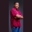Related Tags

swift
communitycreator

# How to get the highest value or upper bound of a range in SwiftTheodore Kelechukwu Onyejiaku

### Overview

We can use the upperBound property of a Range instance to know the upper bound or the highest value of a range in Swift.

### Syntax

range.upperBound


### Return value

The value returned is an integer value that represents the upper bound of a range.

### Code

// create some ranges
let range1 = 1 ..< 6   // 1 to 5
let range2 = 10 ..< 50 // 10 to 49
let range3 = 0 ..< 0   // empty
let range4 = 3 ..< 3   // empty

// get the upper bounds of ranges
print(range1.upperBound)
print(range2.upperBound)
print(range3.upperBound)
print(range4.upperBound)
Get the upper bound of a range in Swift

### Explanation

• Lines 2–5: We create Range instances. Some are empty ranges.
• Lines 8–11: We used upperBound to get the upper bounds of the ranges and printed the values to the console.

RELATED TAGS

swift
communitycreator

CONTRIBUTORTheodore Kelechukwu Onyejiaku
RELATED COURSES

View all Courses

Keep Exploring

Learn in-demand tech skills in half the time